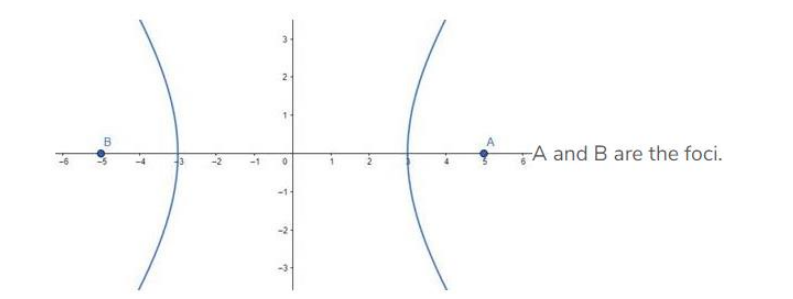# Find the equation of the hyperbola whose foci are (±5, 0) and the conjugate`
Question:

Find the equation of the hyperbola whose foci are (±5, 0) and the conjugate axis is of the length 8. Also, find its eccentricity.

Solution:

Given: Foci are (±5, 0), the conjugate axis is of the length 8

Need to find: The equation of the hyperbola and eccentricity.

Let, the equation of the hyperbola be:

$\frac{x^{2}}{a^{2}}-\frac{y^{2}}{b^{2}}=1$

The conjugate axis is of the length 8, i.e., $2 b=8$

Therefore, $b=4$

The foci are given at $(\pm 5,0)$That means, $\mathrm{ae}=5$, where $\mathrm{e}$ is the eccentricity.

We know that,

$e=\sqrt{1+\frac{b^{2}}{a^{2}}}$

Therefore,

$\Rightarrow \sqrt[a]{1+\frac{b^{2}}{a^{2}}}=5$

$\Rightarrow \sqrt{1+\frac{b^{2}}{a^{2}}}=\frac{5}{a}$

$\Rightarrow 1+\frac{b^{2}}{a^{2}}=\frac{25}{a^{2}}$ [Squaring both sides]

$\Rightarrow \frac{b^{2}}{a^{2}}=\frac{25}{a^{2}}-1=\frac{25-a^{2}}{a^{2}}$

$\Rightarrow b^{2}=25-a^{2}$

$\Rightarrow a^{2}=25-b^{2}=25-16=9$

So, the equation of the hyperbola is,

$\frac{x^{2}}{a^{2}}-\frac{y^{2}}{b^{2}}=1 \Rightarrow \frac{x^{2}}{9}-\frac{y^{2}}{16}=1$

Eccentricity, $\mathrm{e}=\sqrt{1+\frac{\mathrm{b}^{2}}{\mathrm{a}^{2}}}=\sqrt{1+\frac{16}{9}}=\sqrt{\frac{25}{9}}=\frac{5}{3}[$ Answer $]$